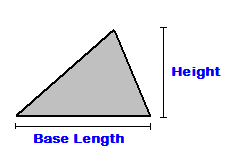## Triangle Area Calculator

Find the area of a triangle with this Triangle Area Calculator.

Base Length:
Height:
Fill in the base length and height of a triangle to find the area.

# How do you find the area of a triangle?

To find the area, use this triangle area formula:
Triangle Area = 1/2 x Base Length x Height

Example: The area of a triangle with a base length of 5 inches
and height of 6 inches is:

Triangle Area = 1/2 x 5 x 6

Calculated out this gives an area of 15 Square Inches.